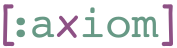## 1    Introduction

A hasher-pair is a pair `[hasher, unhasher]` of functions, where `hasher` is a functions that converts s-expressions into a hash-code, and `unhasher` is a function that converts hash codes back into s-expressions.

For that to work, the `hasher` function needs to store the s-expression as a side-effect, so that the `unhasher` function would be able to retrieve it.

Three components are at play when hashing and unhashing expressions:

1. A serializer/deserializer pair. Standard choices for Clojure can be EDN or Nippy.
2. A hash function. The multihash project gives a versatile and compatible solution.
3. Persistent storage.

## 2    hasher-pair: Construct a Hasher Pair

To construct a hasher-pair, one must supply:

1. A serializer/deserializer pair
2. A hash function (binary array -> hash string)
3. A store/retrieve pair.

For the examples below we use the following mock functions:

``````(defn mock-ser [expr]
[:ser expr])
(defn mock-deser [bin]
[:deser bin])
(def last-stored (atom nil))
(defn mock-store [hash bin]
(reset! last-stored [hash bin]))
(defn mock-retr [hash]
[:retr hash])
(defn mock-hash [bin]
[:hash bin])
(defn mock-unhash [hash])

(def hpair (hasher-pair [mock-ser mock-deser] mock-hash [mock-store mock-retr]))``````
``hpair => vector?``

The `hasher` serializes the s-expression and then calls the hash function on it

``````(let [hasher (first hpair)]
(hasher :some-s-expr) => [:hash [:ser :some-s-expr]])``````

As side-effect, hashing stores the serialized content under the hash.

``@last-stored => [[:hash [:ser :some-s-expr]] [:ser :some-s-expr]]``

The `unhasher` retrieves the content by its hash an de-serializes it.

``````(let [unhasher (second hpair)]
(unhasher "abc") => [:deser [:retr "abc"]])``````

## 3    nippy-multi-hasher: Hasher Based on Multihash and Nippy

To fix some of the variables and create a standard way to hash expressions we use an ipfs-compatible has (using the multihash project), and nippy-based serialization.

``````(let [[hasher unhasher] (nippy-multi-hasher [mock-store mock-retr])]
(hasher ..expr..) => ..hashcode..
(provided
(nippy/freeze ..expr.. nil) => ..bin..
(digest/sha2-256 ..bin..) => ..mhash..
(multihash/base58 ..mhash..) => ..hashcode..)
@last-stored => [..hashcode.. ..bin..]
(unhasher ..hashcode..) => ..expr..
(provided
(nippy/thaw [:retr ..hashcode..] nil) => ..expr..))``````

## 4    file-store: Store content into local files

A real-life application will probably store hashed expressions to some networked storage like Amazon's S3. We provide here storage that uses local files.

The function `file-store` receives a path to a directory in which it assumes the current process has read and write permissions. This is the `repo` directory, where all objects are to be stored.

``````(def repo (str "/tmp/file-store-repo" (rand-int 1000000)))
(.mkdirs (java.io.File. repo)) ; Create the directory if it does not exist``````

It returns a `[store retr]` pair, accepted by hasher-pair and nippy-multi-hasher.

``````(let [[store retr] (file-store repo)]
(store "abcd" (.getBytes "foo bar" "UTF-8")) => nil
; A file is created
(.exists (java.io.File. (str repo "/abcd"))) => true
(-> (str repo "/abcd") slurp-bytes (String. "UTF-8")) => "foo bar"
; This is basically what retr does...
(-> "abcd" retr (String. "UTF-8")) => "foo bar")``````

## 5    atom-store: Store Inside an Atom

For testing it is often convenient to store hashed content in-memory. `atom-store` uses an `atom` for that.

``````(let [[store retr] (atom-store)]
(store "abcd" (.getBytes "foo bar" "UTF-8")) => nil
(-> "abcd" retr (String. "UTF-8")) => "foo bar")``````

## 6    Integration

Let's create a `nippy-multi-hasher` pair that uses local files for storage.

``(def hpair (nippy-multi-hasher (file-store repo)))``

Now let's hash 100 values into it.

``````(def hashes (let [hasher (first hpair)]
(into {} (for [x (range 100)]
[x (hasher {:number x
:double (* 2 x)})]))))``````

Now we retrieve each value and check it.

``````:integ ;; This runs only with lein integ
(let [unhasher (second hpair)]
(doseq [[key hash] hashes]
(unhasher hash) => {:number key
:double (* 2 key)}))``````